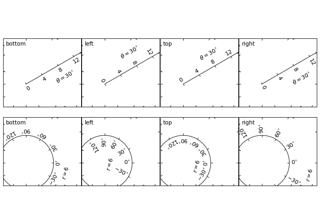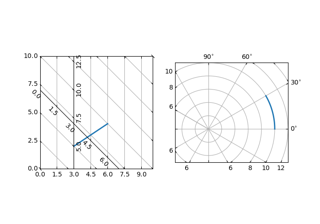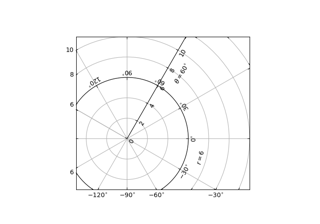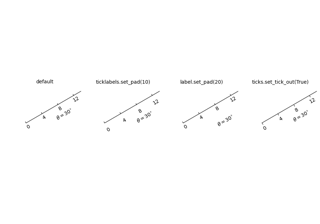mpl_toolkits.axisartist.angle_helper.ExtremeFinderCycle¶

class mpl_toolkits.axisartist.angle_helper.ExtremeFinderCycle(nx, ny, lon_cycle=360.0, lat_cycle=None, lon_minmax=None, lat_minmax=- 90, 90)[source]

This subclass handles the case where one or both coordinates should be taken modulo 360, or be restricted to not exceed a specific range.

Parameters: nx, nyintThe number of samples in each direction. lon_cycle, lat_cycle360 or NoneIf not None, values in the corresponding direction are taken modulo lon_cycle or lat_cycle; in theory this can be any number but the implementation actually assumes that it is 360 (if not None); other values give nonsensical results. This is done by "unwrapping" the transformed grid coordinates so that jumps are less than a half-cycle; then normalizing the span to no more than a full cycle. For example, if values are in the union of the [0, 2] and [358, 360] intervals (typically, angles measured modulo 360), the values in the second interval are normalized to [-2, 0] instead so that the values now cover [-2, 2]. If values are in a range of [5, 1000], this gets normalized to [5, 365]. lon_minmax, lat_minmax(float, float) or NoneIf not None, the computed bounding box is clipped to the given range in the corresponding direction.
__call__(self, transform_xy, x1, y1, x2, y2)[source]

Compute an approximation of the bounding box obtained by applying transform_xy to the box delimited by (x1, y1, x2, y2).

The intended use is to have (x1, y1, x2, y2) in axes coordinates, and have transform_xy be the transform from axes coordinates to data coordinates; this method then returns the range of data coordinates that span the actual axes.

The computation is done by sampling nx * ny equispaced points in the (x1, y1, x2, y2) box and finding the resulting points with extremal coordinates; then adding some padding to take into account the finite sampling.

As each sampling step covers a relative range of 1/nx or 1/ny, the padding is computed by expanding the span covered by the extremal coordinates by these fractions.

__init__(self, nx, ny, lon_cycle=360.0, lat_cycle=None, lon_minmax=None, lat_minmax=- 90, 90)[source]

This subclass handles the case where one or both coordinates should be taken modulo 360, or be restricted to not exceed a specific range.

Parameters: nx, nyintThe number of samples in each direction. lon_cycle, lat_cycle360 or NoneIf not None, values in the corresponding direction are taken modulo lon_cycle or lat_cycle; in theory this can be any number but the implementation actually assumes that it is 360 (if not None); other values give nonsensical results. This is done by "unwrapping" the transformed grid coordinates so that jumps are less than a half-cycle; then normalizing the span to no more than a full cycle. For example, if values are in the union of the [0, 2] and [358, 360] intervals (typically, angles measured modulo 360), the values in the second interval are normalized to [-2, 0] instead so that the values now cover [-2, 2]. If values are in a range of [5, 1000], this gets normalized to [5, 365]. lon_minmax, lat_minmax(float, float) or NoneIf not None, the computed bounding box is clipped to the given range in the corresponding direction.
__module__ = 'mpl_toolkits.axisartist.angle_helper'

Examples using mpl_toolkits.axisartist.angle_helper.ExtremeFinderCycle¶